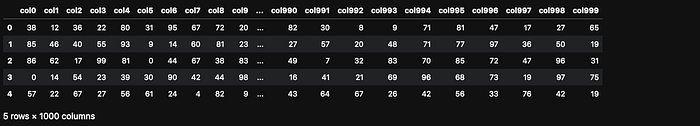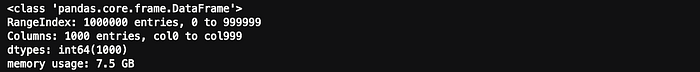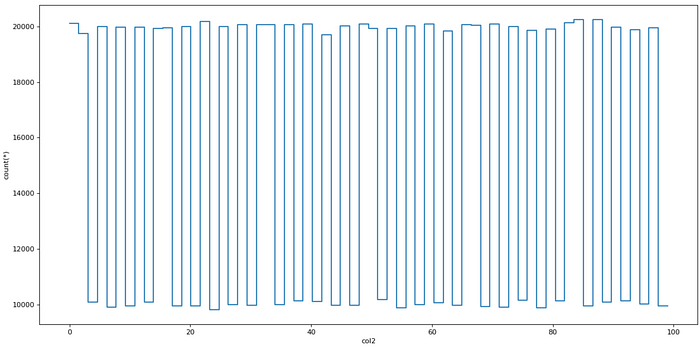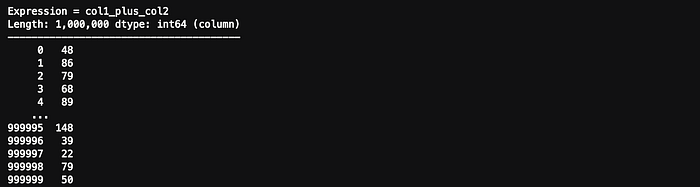# How to Process a DataFrame With Millions of Rows in Seconds

Yet another Python library for Data Analysis that You Should Know About — and no, I am not talking about Spark or Dask

Big Data Analysis in Python is having its renaissance. It all started with NumPy, which is also one of the building blocks behind the tool I am presenting in this article.

In 2006, Big Data was a topic that was slowly gaining traction, especially with the release of Hadoop. Pandas followed soon after with its DataFrames. 2014 was the year when Big Data became mainstream, also Apache Spark was released that year. In 2018 came Dask and other libraries for data analytics in Python.

Each month I find a new Data Analytics tool, which I am eager to learn. It is a worthy investment of spending an hour or two on tutorials as it can save you a lot of time in the long run. It’s also important to keep in touch with the latest tech.

Like Python, it is equally important that you become proficient in SQL. In case you aren’t familiar with it, and you have some money to spare, check out this course: Master SQL, the core language for Big Data analysis.

Big Data Analysis in Python is having its renaissance

```- Intro to Machine Learning with PyTorch
- Deep Learning Nanodegree and more```

# Meet VaexVaex is a high-performance Python library for lazy Out-of-Core DataFrames (similar to Pandas), to visualize and explore big tabular datasets.

It can calculate basic statistics for more than a billion rows per second. It supports multiple visualizations allowing interactive exploration of big data.

What’s the difference between Vaex and Dask?Vaex is not similar to Dask but is similar to Dask DataFrames, which are built on top pandas DataFrames. This means that Dask inherits pandas issues, like high memory usage. This is not the case Vaex.

Vaex doesn’t make DataFrame copies so it can process bigger DataFrame on machines with less main memory.

Both Vaex and Dask use lazy processing. The only difference is that Vaex calculates the field when needed, wherewith Dask we need to explicitly use the compute function.

Data needs to be in HDF5 or Apache Arrow format to take full advantage of Vaex.

How to install Vaex?

To install Vaex is as simple as installing any other Python package:
`pip install vaex`

# Let’s take Vaex to a test driveLet’s create a pandas DataFrame with 1 million rows and 1000 columns to create a big data file.
```import vaex
import pandas as pd
import numpy as np
n_rows = 1000000
n_cols = 1000
df = pd.DataFrame(np.random.randint(0, 100, size=(n_rows, n_cols)), columns=['col%d' % i for i in range(n_cols)])First few lines in a Pandas Dataframe (image made by author)

How much main memory does this DataFrame use?
`df.info(memory_usage='deep')`Let’s save it to disk so that we can read it later with Vaex.
```file_path = 'big_file.csv'
df.to_csv(file_path, index=False)```

We wouldn’t gain much by reading the whole CSV directly with Vaex as the speed would be similar to pandas. Both need approximately 85 seconds on my laptop.

We need to convert the CSV to HDF5 (the Hierarchical Data Format version 5) to see the benefit with Vaex. Vaex has a function for conversion, which even supports files bigger than the main memory by converting smaller chunks.

If you cannot open a big file with pandas, because of memory constraints, you can covert it to HDF5 and process it with Vaex.
`dv = vaex.from_csv(file_path, convert=True, chunk_size=5_000_000)`

This function creates an HDF5 file and persists it to disk.

What’s the datatype of dv?
```type(dv)
# output
vaex.hdf5.dataset.Hdf5MemoryMapped```

Now, let’s read the 7.5 GB dataset with Vaex — We wouldn’t need to read it again as we already have it in dv variable. This is just to test the speed.
`dv = vaex.open('big_file.csv.hdf5')`

Vaex needed less than 1 second to execute the command above. But Vaex didn’t actually read the file, because of lazy loading, right?

Let’s force to read it by calculating a sum of col1.
```suma = dv.col1.sum()
suma
# Output
# array(49486599)```

I was really surprised by this one. Vaex needed less than 1 second to calculate the sum. How is that possible?

Opening such data is instantaneous regardless of the file size on disk. Vaex will just memory-map the data instead of reading it in memory. This is the optimal way of working with large datasets that are larger than available RAM.

Plotting

Vaex is also fast when plotting data. It has special plotting functions plot1d, plot2d and plot2d_contour.
`dv.plot1d(dv.col2, figsize=(14, 7))`Plotting with Vaex (image made by author)

Virtual columns

Vaex creates a virtual column when adding a new column, — a column that doesn’t take the main memory as it is computed on the fly.
```dv['col1_plus_col2'] = dv.col1 + dv.col2
dv['col1_plus_col2']```The virtual column in Vaex (image made by author)

Efficient filtering

Vaex won’t create DataFrame copies when filtering data, which is much more memory efficient.
```dvv = dv[dv.col1 > 90]
```

# Aggregations

Aggregations work slightly differently than in pandas, but more importantly, they are blazingly fast.

Let’s add a binary virtual column where col1 ≥ 50.
`dv['col1_50'] = dv.col1 >= 50`

Vaex combines group by and aggregation in a single command. The command below groups data by the “col1_50” column and calculates the sum of the col3 column.
```dv_group = dv.groupby(dv['col1_50'], agg=vaex.agg.sum(dv['col3']))
dv_group```Aggregations in Vaex (image made by author)

Joins

Vaex joins data without making memory copies, which saves the main memory. Pandas users will be familiar with the join function:
```dv_join = dv.join(dv_group, on=’col1_50')
```

# Conclusion

In the end, you might ask: Should we simply switch from pandas to Vaex? The answer is a big NO.

Pandas is still the best tool for data analysis in Python. It has well-supported functions for the most common data analysis tasks.

When it comes to bigger files, pandas might not be the fastest tool. This is a great place for Vaex to jump in.

Vaex is a tool you should add to your Data Analytics toolbox.

When working on an analysis task where pandas is too slow or simply crashes, pull Vaex out of your toolbox, filter out the most important entries and continue the analysis with pandas.

“How to Process a DataFrame With Millions of Rows in Seconds”
– Roman Orac

### Related Stories

Nov 25, 2021

#### 5 Tips To Ace Your Job Interview For A Data Scientist Opening

5 Tips To Ace Your Job Interview For A Data Scientist Opening.PNG 795.94 KBImage SourceAspiring data scientists have a bright future ahead of them....By Daniel Morales
Nov 12, 2021

#### When to Avoid Deep LearningBy Matt Przybyla
Oct 16, 2021

#### 6 Advanced Statistical Concepts in Data Science

The article contains some of the most commonly used advanced statistical concepts along with their Python implementation.In my previous articles Be...By Nagesh Singh Chauhan